Next: 4.4 Effective Mass of Up: 4. Quantum Confinement and Previous: 4.2 Effective Mass of

# 4.3 Quantization in UTB Films for Primed Subbands

As pointed out before a shear strain component in thedirection does not affect the primed valleys along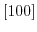and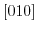direction, except for a small shift of the minimum . However, the linear combination of bulk bands method gained with the empirical pseudo-potential calculations  and calculations of the primed subbands based on the density functional theory (DFT)  uncover the relationship of the transport effective masses on the silicon film thickness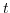. Here we analyze the dependence of the primed subbands effective mass via the two-band k.p Hamiltonian utilized before (4.1). At first we have to derive analogously to the unprimed subbands an analytical expression for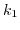as a function ofand vice versa: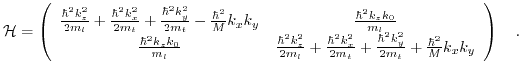(4.27)

Starting with the transformation to dimensionless form according to: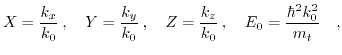(4.28)

and some further rearrangements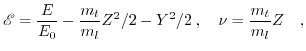(4.29)

the eigenvalue problem takes the following form: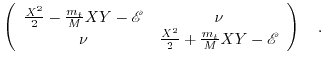(4.30)

Setting the determinant of (4.30) to zero allows to obatin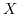as a function of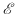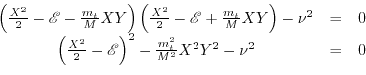(4.31)

Like before for the unprimed subbands, the obtained fourth order equation,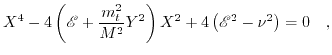(4.32)

can be reformulated into two second order equations:(4.33)

The identities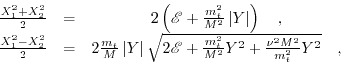(4.34)

allow to introduce a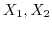dependence in (4.33) and formulate the problem as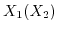and vice versa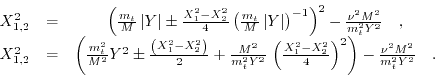(4.35)

Soas a function of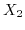is described by the following equation: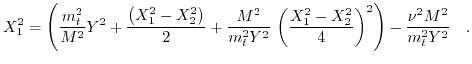(4.36)

Substituting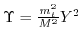into (4.36) results in: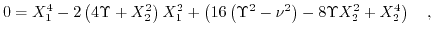(4.37)

and enables the derivation ofas a function of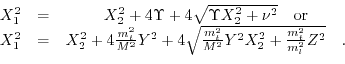(4.38)

In order to obtain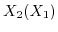the minus branch of (4.35) is used: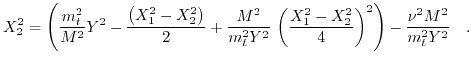(4.39)

After analogous treatment of (4.39) the relation ofas a function ofis derived: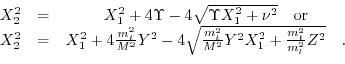(4.40)

The corresponding scaled energy dispersion relation is given by: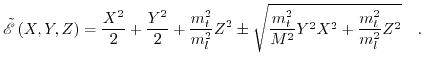(4.41)Next: 4.4 Effective Mass of Up: 4. Quantum Confinement and Previous: 4.2 Effective Mass of

T. Windbacher: Engineering Gate Stacks for Field-Effect Transistors